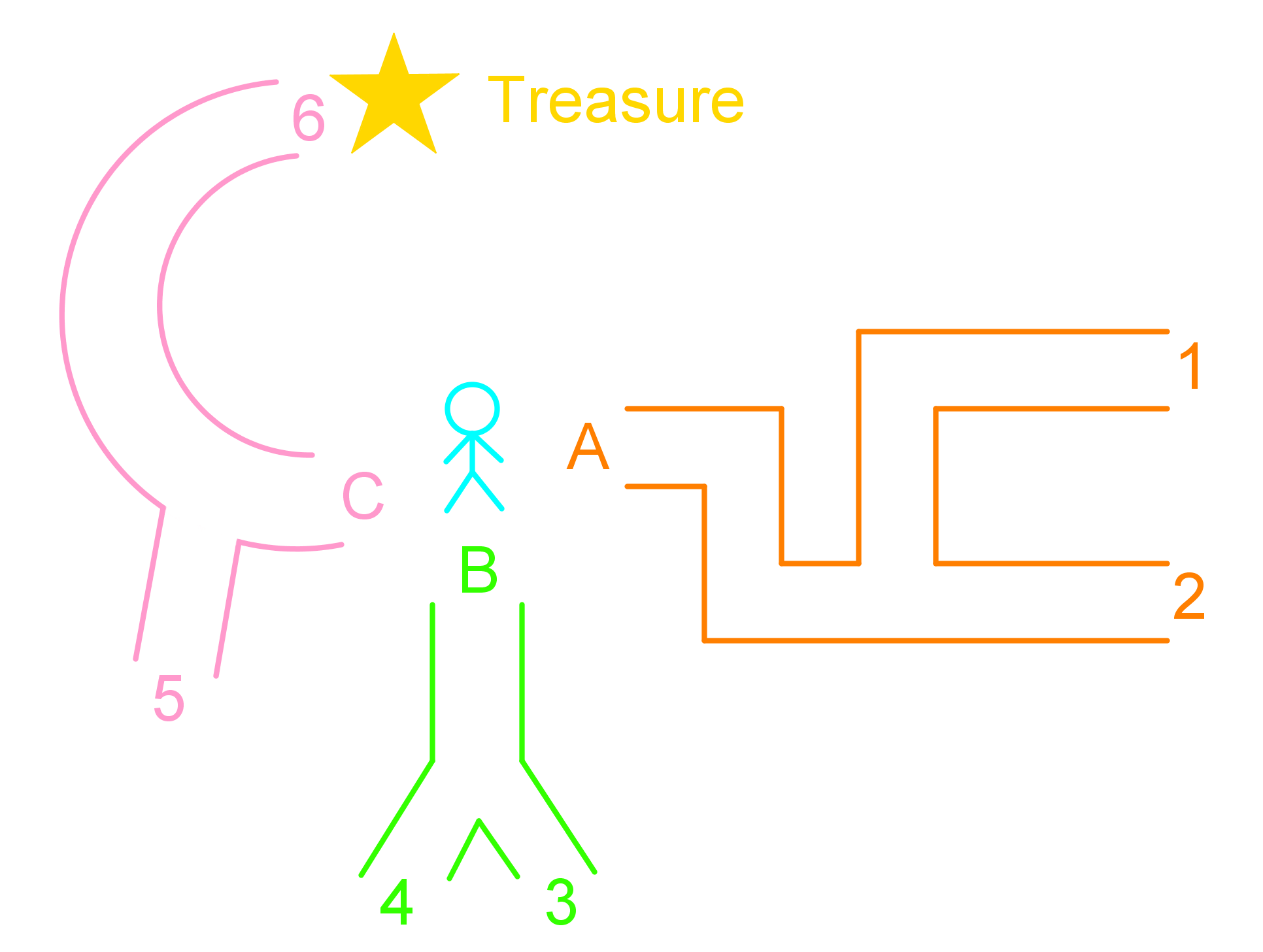# Probability of independent events

### Probability of independent events

Similar to the section about organizing outcomes, in this section we practice determining the outcomes of two independent events along with organizing these outcomes using tables and tree diagrams. In this section, we are also going to learn how to determine the number of possible outcomes in two independent events. In addition to finding the number of possible outcomes, in this section we practice finding the number of favorable outcomes in two independent events. Finally, in this section we use tables and tree diagrams to solve probability problems.

#### Lessons

• Introduction
Differences between independent events and dependent events

• 1.
Sam is hunting a treasure. He is equally likely to travel along any pathway. His trip ends at 1, 2, 3, 4, 5, or 6.a)
What is the probability that Sam finds the treasure?

b)
What is the probability that Sam does not find the treasure?

• 2.
Claire rolls two 6-sided dice.
a)
If Claire rolls two threes or two fives, then she will buy herself a donut. How many favorable outcomes are there?

b)
What is the probability of getting at least one odd number?

c)
If she rolls the two dice and adds the numbers together, how many times will the sum be 7?

• 3.
Anna has 18 clothes in her closet. She has 6 shirts, 5 tees, 4 tanks and 3 sweaters. If she is taking 1 top from the closet randomly, what is the probability that:
a)
She will take out a tee?

b)
She will take out a shirt or a sweater?

c)
She will not take out a shirt or a tank?

d)
She will take out a sweater and a tee, if she takes out 1 item of cloth, puts it back and takes out a second one?